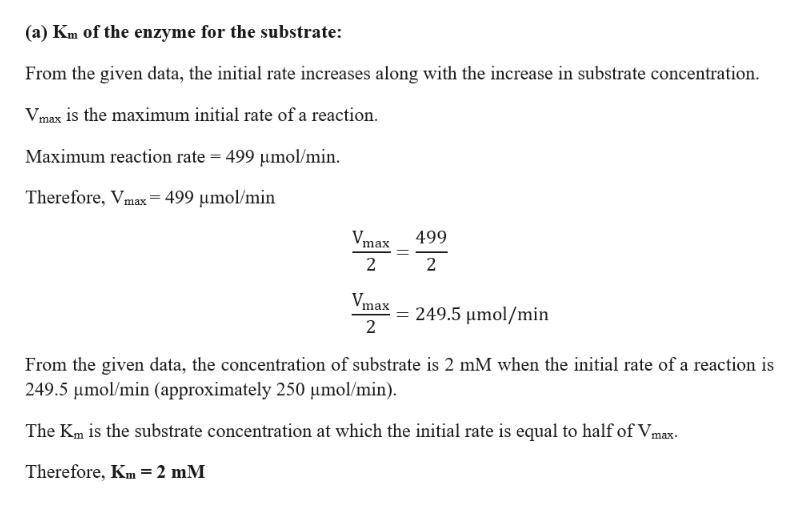# The turnover number for an enzyme is known to be 5000min-1. Given the following set of data, Substrate concentration(mM)1, 2, 4, 6, 100, 1,000Initial Rate(micromol/min)167, 250, 334, 376, 498, 499 a) What is the Km of the enzyme for the substrate? (do this without using a calculator)b) What is the total amount of enzyme present in the assay?

Question
306 views

The turnover number for an enzyme is known to be 5000min-1. Given the following set of data,

Substrate concentration(mM)

1, 2, 4, 6, 100, 1,000

Initial Rate(micromol/min)

167, 250, 334, 376, 498, 499

a) What is the Kof the enzyme for the substrate? (do this without using a calculator)

b) What is the total amount of enzyme present in the assay?

check_circle

Step 1

Enzyme kinetics is defined as the study of chemical reactions which are catalyzed by enzymes. Km is the Michaelis constant and is very essential in determining of enzyme-substrate interaction.

Step 2help_outlineImage Transcriptionclose(a) Km of the enzyme for the substrate: From the given data, the initial rate increases along with the increase in substrate concentration VImax is the maximum initial rate of a reaction. Maximum reaction rate 499 umol/min Therefore, Vmax=499 umol/min Vmax 499 2 2 Vmax 249.5 umol/min 2 From the given data, the concentration of substrate is 2 mM when the initial rate of a reaction is 249.5 umol/min (approximately 250 umol/min) The Km is the substrate concentration at which the initial rate is equal to half of Vmax- Therefore, Km=2 mM fullscreen

### Want to see the full answer?

See Solution

#### Want to see this answer and more?

Solutions are written by subject experts who are available 24/7. Questions are typically answered within 1 hour.*

See Solution
*Response times may vary by subject and question.
Tagged in

### Other